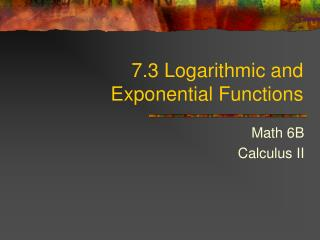DownloadDownload Presentation7.3 Logarithmic and Exponential Functions

# 7.3 Logarithmic and Exponential Functions

Download Presentation## 7.3 Logarithmic and Exponential Functions

- - - - - - - - - - - - - - - - - - - - - - - - - - - E N D - - - - - - - - - - - - - - - - - - - - - - - - - - -
##### Presentation Transcript

1. 7.3 Logarithmic and Exponential Functions Math 6B Calculus II

2. Exponential Functions • The graph of y =bx, where b > 1 • The graph of y =bx, where 0 < b < 1

3. Inverse Relations for Exponential and Logarithmic Functions • For any base b > 0, with , the following inverse relation hold. • Solving Logarithmic and Exponential Equations.

4. The Derivative of bx • We will use logarithmic differentiation to find dy/dx where y = bx • The end resultwe get is:

5. Exponential Integral • Exponential Integral of bx:

6. The Power Rule • If n is any real number and f (x) = xn, then f ‘(x) = nxn-1

7. The Evolution of logbx